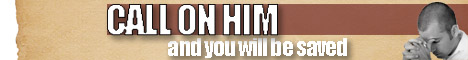Note:  Do not rely on this information. It is very old.

# Decimal Fractions

Decimal Fractions, in arithmetic, a system of expressing fractional portions of unity by means of a series of fractions, the denominators of which are powers of ten. Thus, 7/16 may be represented as 4/10 + 3/100 + 7/1000 + 5/10000, or in the usual abreviated form .4375, the position of each digit after the decimal point telling us the magnitude of the denominator of the corresponding fraction. Any number may bo so expressed, though it sometimes happens that simple fractions demand a long series of decimals. Thus, 5/128 is equivalent to .039375, and 13/99 is .13131313 ..., the digits 1 and 3 alternating forever: that is to say, certain fractions like the latter demand an infinite series of decimals to be exactly expressed thereby. Such recurrence is signified by placing dots over the first and last figures of the sot that have to be repeated; the above recurring decimal is written .13 [with dots over the 1 and the 3], the decimal .103103103 ... is written .103 [with dots over the 1 and the 3] and 4.35732732732 ... is written 4.35732 [with dots over the 7 and the 2]. There are simple rules for the conversion of vulgar fractions into decimals and for the reverse process. Those rules are based on elementary considerations of fractions, and need not here be given. The use of decimals has distinct advantages; the processes of addition and subtraction may be effected much more readily with them than with vulgar fractions; different magnitudes may be compared by simple inspection when expressed as decimals. Thus, the relative values of the fractions 22/7 and 355/113 are readily seen when they are brought to the forms 3.14285 ... and 3.14159 ... respectively. In multiplication of decimal fractions one operation alone is necessary, whereas with vulgar fractions two are requisite. Nevertheless the decimal operations are sometimes more tedious, and as stated above simple ratios frequently require to be expressed as complex decimals.